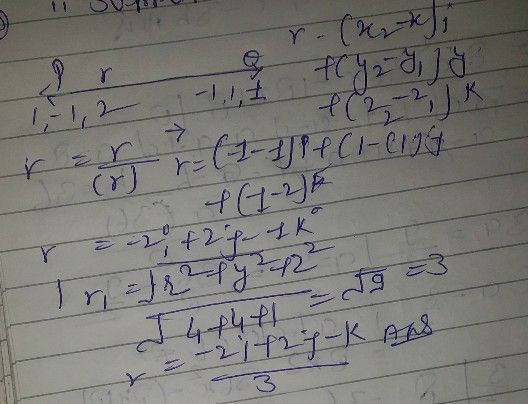Symbol
Problem$39$ Find the directional derivative of $f\left(x,y\right)=.1^{2}+y^{2}$ at the YV point $P_{0}\left(1_{,1}\right)$ in the diréction of the unit vector u shown in Figure $11.35$ $11$ $l=coac+6w$ $\dfrac {\pi } {6}$ $b$ $P$ $\left(0$
Calculus
Search count: 120
SolutionQanda teacher - Devkiranplease rate in 5 star